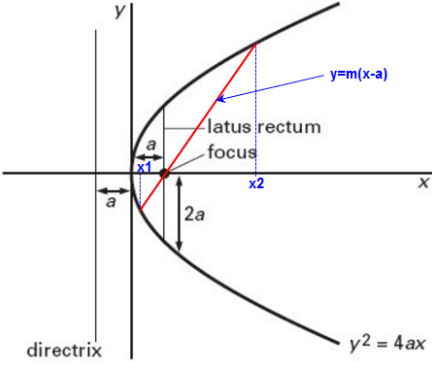# If two tangents drawn from a point P to the parabola are at right angles, then the locus of P is Option 1) Option 2) Option 3) Option 4)

P Plabita

As we learnt in

Equation of tangent -

- wherein

Tangent at

and

Standard equation of parabola -

- whereinLocus of perpendicular tangents is the directrix.

so, x = -a

i.e. x = -1

Option 1)

This option is incorrect.

Option 2)

This option is incorrect.

Option 3)

This option is correct.

Option 4)

This option is incorrect.

Exams
Articles
Questions